#### Obtaining a discrete planning problem

Once the grid and neighborhoods have been defined, a discrete planning problem is obtained. Figure 5.14 depicts the process for a problem in which there are nine Sukharev grid points in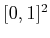. Using-neighborhoods, the potential edges in the search graph,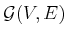, appear in Figure 5.14a. Note that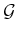is a topological graph, as defined in Example 4.6, because each vertex is a configuration and each edge is a path. If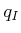and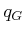do not coincide with grid points, they need to be connected to some nearby grid points, as shown in Figure 5.14b. What grid points shouldandbe connected to? As a general rule, if-neighbors are used, then one should try connectingandto any grid points that are at least as close as the furthest-neighbor from a typical grid point.

Usually, all of the vertices and edges shown in Figure 5.14b do not appear inbecause some intersect with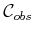. Figure 5.14c shows a more typical situation, in which some of the potential vertices and edges are removed because of collisions. This representation could be computed in advance by checking all potential vertices and edges for collision. This would lead to a roadmap, which is suited for multiple queries and is covered in Section 5.6. In this section, it is assumed thatis revealed on the fly'' during the search. This is the same situation that occurs for the discrete planning methods from Section 2.2. In the current setting, the potential edges ofare validated during the search. The candidate edges to evaluate are given by the definition of the-neighborhoods. During the search, any edge or vertex that has been checked for collision explicitly appears in a data structure so that it does not need to be checked again. At the end of the search, a path is found, as depicted in Figure 5.14d.

Steven M LaValle 2020-08-14